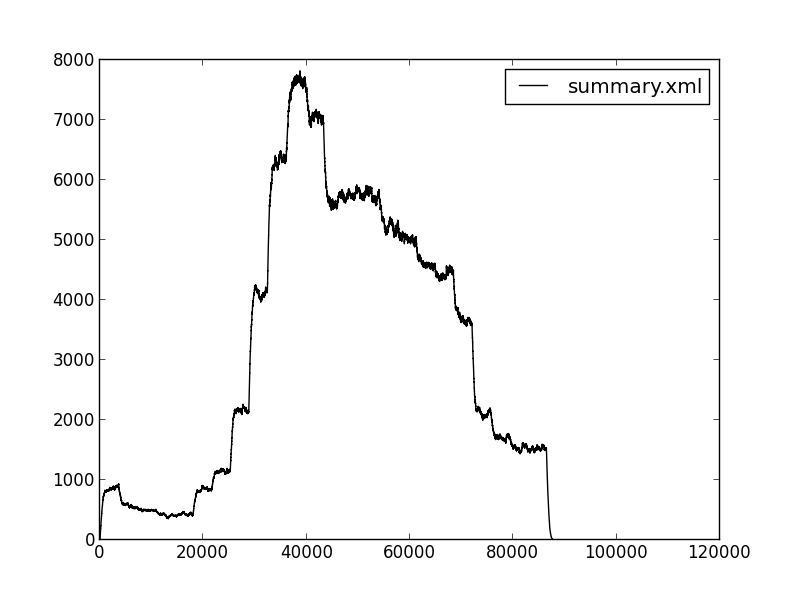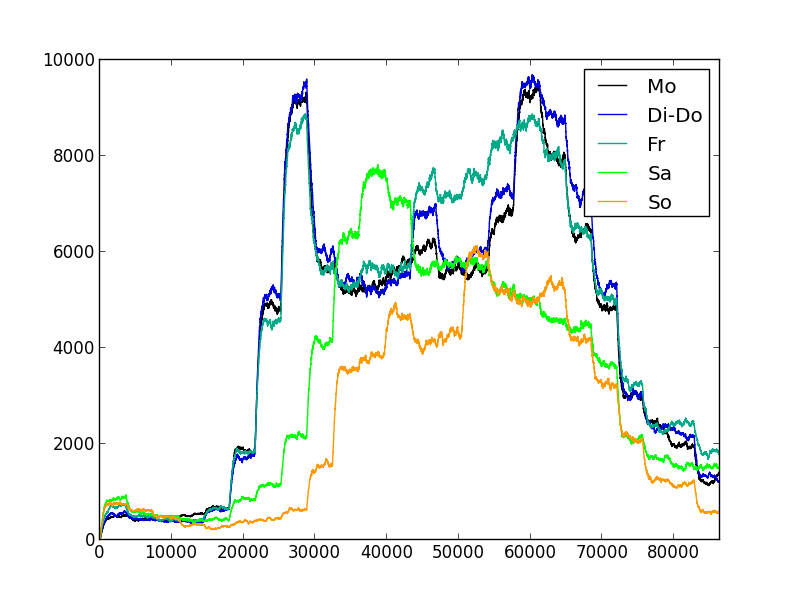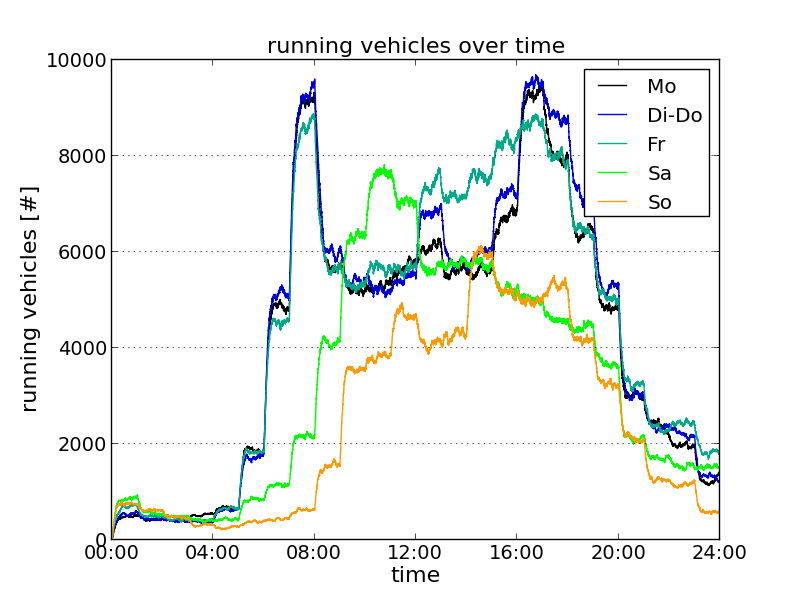Tutorials/Plotting Cookbook

In the following, You will find some examples for plotting results obtained from SUMO.

## Number of Vehicles within the Simulation over Time#

Let's assume You want to show the number of vehicles running through your simulation over time. You'll need the summary-output that contains the number of vehicles within the simulation for each simulation time step.

If Your summary-output is named "summary.xml", You may show the number of running vehicles using

``````python plot_summary.py -i summary.xml
``````

as `running`, the measurement You want to plot, is the default. The following image will be shown:Now, let's assume You want to compare the number of running vehicles from different runs. The example below uses summary-outputs from runs that cover different types of days of a week (mo\summary.xml: Monday, dido\summary.xml: Tuesday-Tursday, etc.). As we want to show only a single day, we limit the x-axis to [0,86400] (86400: seconds in a day) using --xlim 0,86400 and we also save the figure into the file "summary_running_all.png" using -o summary_running_all.png. To know what is shown, we replace the file names in the legend by the week day types using -l Mo,Di-Do,Fr,Sa,So.

``````python plot_summary.py -i mo\summary.xml,dido\summary.xml,fr\summary.xml,sa\summary.xml,so\summary.xml \
-l Mo,Di-Do,Fr,Sa,So -o summary_running_all.png --xlim 0,86400
``````Ok, now we'll make it professional by adding labels and titles, proper ticks, using a different format for the simulation time than seconds, etc.

``````python plot_summary.py -i mo\summary.xml,dido\summary.xml,fr\summary.xml,sa\summary.xml,so\summary.xml \
-l Mo,Di-Do,Fr,Sa,So --xlim 0,86400 --ylim 0,10000 -o sumodocs/summary_running.png --yticks 0,10001,2000,14 \
--xticks 0,86401,14400,14 --xtime1 --ygrid --ylabel "running vehicles [#]" --xlabel "time" \
--title "running vehicles over time" --adjust .14,.1
``````The summary-output contains further measures that can be visualised in the same way, among them `loaded`, `inserted`, `waiting`, or `ended`. Please consult the summary-output documentation for a complete list.

Further information can be found at: